• 文章目录1. 加载宏包2. plot 函数的语法plot([x], y, [fmt], **kwargs)3. 一张图画好几条线 1. 加载宏包 使用 matplotlib 包...# 导入 matplotlib 中的 plot， 并命名为常用名 plt import matplotlib.pyplot as p...


文章目录
1. 加载宏包2. plot 函数的语法plot([x], y, [fmt], **kwargs)

3. 一张图画好几条线

1. 加载宏包
使用 matplotlib 包画图时，我们一般加载里面的 pyplot，并命名为 plt，然后使用 plot 函数画图。
# 导入 matplotlib 中的 plot， 并命名为常用名 plt
import matplotlib.pyplot as plt

例如，下面的代码画出正弦函数

y

=

s

i

n

(

x

)

y=sin(x)

的图形。
import matplotlib.pyplot as plt
import numpy as np

# 生成数据
x = np.arange(0, 10, 0.1) # 横坐标数据为从0到10之间，步长为0.1的等差数组
y = np.sin(x) # 纵坐标数据为 x 对应的 sin(x) 值

# 生成图形
plt.plot(x, y)

# 显示图形
plt.show()

图形显示：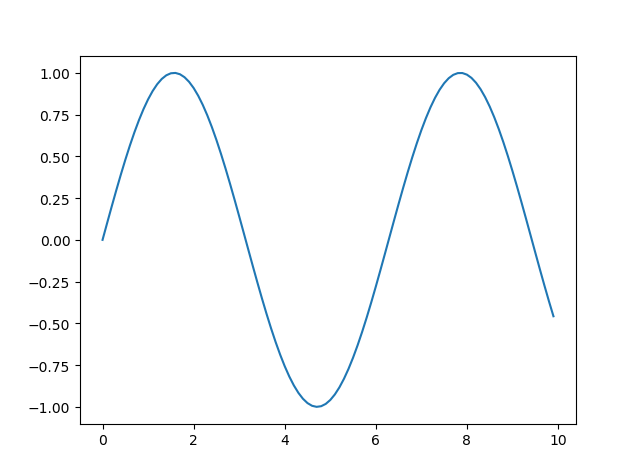利用plot函数，我们可以对图形进行更多精细的设置，官方的详细文档可以参看：https://matplotlib.org/3.1.1/api/_as_gen/matplotlib.pyplot.plot.html。
2. plot 函数的语法
Plot 函数的基本语法是：

plot([x], y, [fmt], **kwargs)

含义[x]可选参数，横坐标轴数据y纵坐标轴数据[fmt]可选参数，字符串，定义图形的基本样式：颜色，点形，线形**Kwargs不定长的关键字参数，用字典形式设置图形的其他属性
[fmt] 的常用代码（包括颜色代码、点形代码、线形代码），由下面的表所示。 颜色：
代码颜色‘b’蓝色‘g’绿色‘r’红色‘y’黄色‘k’黑色‘w’白色
点形：
代码点形‘.’圆点形‘o’实心圆‘*’星号‘+’加号‘x’叉号
线形：
代码线形‘-’实线‘–’虚线‘-.’折线‘:’点线
**Kwargs 的常用设置包括线条的粗细 linewidth，图像标签 label 等。下面一些 plot 函数的代码展示了 [x]，[fmt]，**Kwargs 的一些可选用法：
>>> plot(x, y)        # 根据横坐标数据 x 与纵坐标数据 y 画图，采用默认的颜色、点形与线性
>>> plot(y)           # 据纵坐标数据 y 画图，横坐标数据默认为从 0 到 N-1，步长为 1 的等差数组
>>> plot(x, y, 'bo')  # 颜色为蓝色('b')、点形为圆('o')
>>> plot(y, 'g-.')     # 颜色为绿色('g')，线型为折线('-.')
>>> plt.plot(x, y, 'yo:', label='y=sin(x)', linewidth=2) # 颜色为黄色('y')，点形为圆形('o')，线形为虚线(':')，lable 内容为 'y=sin(x)', 线条宽度为 2

通过设置线型形状，就可以画折线图了。
如果我们想自定义坐标轴的标题，坐标轴的刻度，坐标轴刻度的范围，设置图形标题，添加图例时，可以通过设置 pyplot 函数中的 xlable（横坐标轴标题）, ylabel（纵坐标轴标题）, xticks（横坐标轴刻度），yticks（纵坐标轴刻度），title（图形标题）, grid（显示网格），legend（显示图例）等属性来实现。经过自定义设置，对上图的代码进行一下修改：
import matplotlib.pyplot as plt
import numpy as np

# 这两行代码使得 pyplot 画出的图形中可以显示中文
plt.rcParams['font.sans-serif'] = ['SimHei']
plt.rcParams['axes.unicode_minus'] = False

# 生成数据
x = np.arange(0, 10, 0.5)
y = np.sin(x)

# 生成图形
plt.plot(x, y, 'go:', label='y=sin(x)', linewidth=2) # 颜色绿色，点形圆形，线性虚线，设置图例显示内容，线条宽度为2

plt.ylabel('y') # 横坐标轴的标题
plt.xlabel('x') # 纵坐标轴的标题
plt.xticks(np.arange(0, 11, 1)) # 设置横坐标轴的刻度为 0 到 10 的数组
plt.ylim([-2, 2]) # 设置纵坐标轴范围为 -2 到 2
plt.legend() # 显示图例, 图例中内容由 label 定义
plt.grid() # 显示网格
plt.title('我的第一个 Python 图形') # 图形的标题

# 显示图形
plt.show()

生成图形：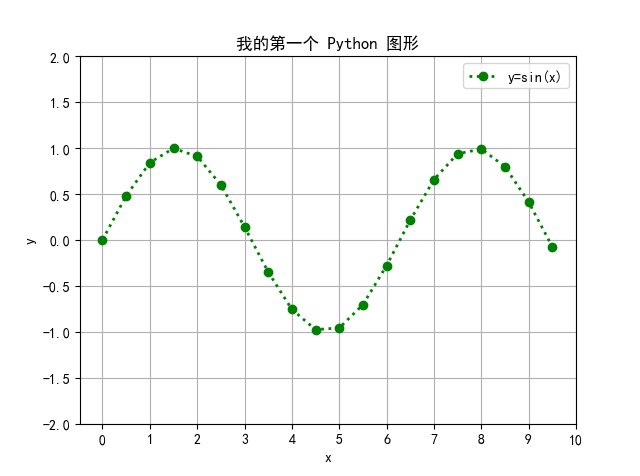3. 一张图画好几条线
下表是我国近10年的 GDP 增长率，以及三大产业在近10年的增长率。
时间GDP增长率第一产业增长率第二产业增长率第三产业增长率2009年9.4410.39.62010年10.64.312.79.72011年9.64.210.79.52012年7.94.58.482013年7.83.888.32014年7.34.17.47.82015年6.93.96.28.22016年6.73.36.37.72017年6.845.97.92018年6.63.55.87.6
在画图时，横坐标轴数据为年份，纵坐标轴数据分别为 GDP 增长率，第一产业增长率，第二产业增长率，第三产业增长率。为了将四个纵坐标轴数据显示在一个图形上，可以用四个 plot 函数进行划线。Python 画图的代码为：
import matplotlib.pyplot as plt

# 这两行代码解决 plt 中文显示的问题
plt.rcParams['font.sans-serif'] = ['SimHei']
plt.rcParams['axes.unicode_minus'] = False

# 输入纵坐标轴数据与横坐标轴数据
gdp_rate = [9.4, 10.6, 9.6, 7.9, 7.8, 7.3, 6.9, 6.7, 6.8, 6.6]
first_industry_rate = [4.0, 4.3, 4.2, 4.50, 3.8, 4.1, 3.9, 3.3, 4.0, 3.5]
second_industry_rate = [10.3, 12.7, 10.7, 8.4, 8.0, 7.4, 6.2, 6.3, 5.9, 5.8]
third_industry_rate = [9.6, 9.7, 9.5, 8.0, 8.3, 7.8, 8.2, 7.7, 7.9, 7.6]
years = [2009, 2010, 2011, 2012, 2013, 2014, 2015, 2016, 2017, 2018]

# 4 个 plot 函数画出 4 条线，线形为折线，每条线对应各自的标签 label
plt.plot(years, gdp_rate, '.-', label='GDP增长率')
plt.plot(years, first_industry_rate, '.-', label='第一产业增长率')
plt.plot(years, second_industry_rate, '.-', label='第二产业增长率')
plt.plot(years, third_industry_rate, '.-', label='第三产业增长率')

plt.xticks(years)  # 设置横坐标刻度为给定的年份
plt.xlabel('年份') # 设置横坐标轴标题
plt.legend() # 显示图例，即每条线对应 label 中的内容
plt.show() # 显示图形

图形显示效果：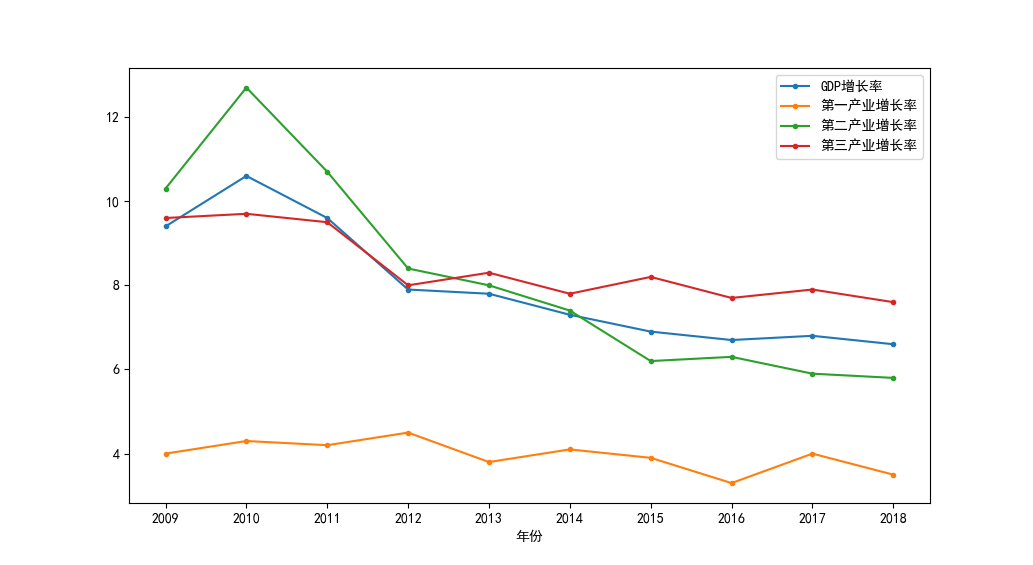展开全文python pyplot
• matplotlib.pyplot.scatter(x, y, s=None, c=None, marker=None, cmap=None, norm=None, vmin=None, vmax=None, alpha=None, linewidths=None, verts=None, edgecolors=None, *, plotnonfinite=F...
Scatter（）函数描述
官网函数 matplotlib.pyplot.scatter(x, y, s=None, c=None, marker=None, cmap=None, norm=None, vmin=None, vmax=None, alpha=None, linewidths=None, verts=None, edgecolors=None, *, plotnonfinite=False, data=None, **kwargs) x,y:输入打点数据。 s:标记大小？ c:颜色。？和color区别？ maker：标记的符号。更多符号符号 alpha：制定透明度，范围：0-1. linewidths：线宽，标记边缘的线宽。 edgecolors：边缘颜色，
1.画布的绘制
方法1：
 测试绘制画布区域
fig = plt.figure(num='测试用图', figsize=(10, 5), dpi=50,
facecolor='yellow', edgecolor='yellow', frameon=True)
# num:画布的标题命名 常用
# figsiez:指定figure的宽和高，英寸。
# dpi:指定分辨率
# facecolor：背景颜色
# edgecolor：边框颜色
# frameon:是否显示边
plt.plot(2, 2, 'o', color='red')
plt.plot(2, 2, 'o', color='black')方法二：
fig, axes = plt.subplots(2, 2, num='测试用图1', figsize=(10, 5), dpi=50,
facecolor='yellow', edgecolor='yellow', frameon=True)
#2,2指定nrows和ncols,可以不写，直接写成2，2
#通常只需要用axes。直接指定每个子图的画法。
axes[0, 0].plot(2, 2, 'o', color='black')
axes[0, 1].plot(2, 2, 'o', color='red')
plt.show()2.XY轴样式的更改
fig, axes = plt.subplots(num='测试用图1', figsize=(10, 10), dpi=50,
facecolor='yellow', edgecolor='yellow', frameon=True)
# 2,2指定nrows和ncols,可以不写，直接写成2，2
axes.plot([-1, 2, 4], [0, 2, 4], '-o', color='red')
plt.title('图形1', fontsize=30, color='red')
plt.xlabel('x坐标轴', fontsize=30, color='k')
plt.ylabel('y坐标轴', fontsize=30, color='k')
plt.title('图形1', fontsize=30, Color='k')
# 改变XY坐标轴的操作对象
ax = plt.gca()
# 改变XY坐标轴的外边框
ax.spines['right'].set_color('none')
ax.spines['top'].set_color('none')
ax.xaxis.set_ticks_position('bottom')
ax.yaxis.set_ticks_position('left')
# 改变XY零点坐标的位置
ax.spines['bottom'].set_position(('data', 0))  # 将X轴的数据绑定到Y轴的0点上
ax.spines['left'].set_position(('data', 0))  # 将Y轴的数据板顶到X轴的0点上

plt.xticks(list(range(-10, 5, 1)), fontsize=25, rotation=0)
plt.yticks(list(range(-10, 5, 1)), fontsize=25, rotation=0)
plt.xlim(-2, 4)
plt.ylim(-2, 4)
# 对于改变字体,颜色的操作对象需重新调用一次,否则会绘制出错.
# axes.xaxis.grid(True, which='major')
# xticks(ticks,[labels],**kwargs)
# ticks：数组类型，用于设置X轴刻度间隔
# [labels]：数组类型，用于设置每个间隔的显示标签
# **kwargs：用于设置标签字体倾斜度和颜色等外观属性
plt.grid()  # 网格绘制
plt.show()展开全文• Matplotlib的画图功能是数据分析里面非常好用的一款工具，这款工具可以做到将庞杂的实验数据用以图表的形式简单直观的显示出来,由于之后的学习过程中会频频使用到数据进行实验,掌握了这款工具之后的学习可以便利很多...
Matplotlib的画图功能是数据分析里面非常好用的一款工具，这款工具可以做到将庞杂的实验数据用以图表的形式简单直观的显示出来,由于之后的学习过程中会频频使用到数据进行实验,掌握了这款工具之后的学习可以便利很多,这里简单记录了一些plot的命令

首先要导入我们接下来要用到的库
# -*- coding=utf8 -*-
import numpy as np
import matplotlib.pyplot as plt
尝试运行构建一个简单的图表出来
plt.xlabel('x1') #将坐标系x轴命名为x1
plt.ylabel('y1') #将坐标系y轴命名为y1
plt.axis([0,10,0,100]) #定义x轴的范围为0,10 定义y轴的范围为0,100
plt.savefig('/..../myfirst_plt', dpi = 600) #保存图表.单引号内为保存地址,默认png,dpi为分辨率
plt.show() #显示你的图表
运行之后图片效果显示如下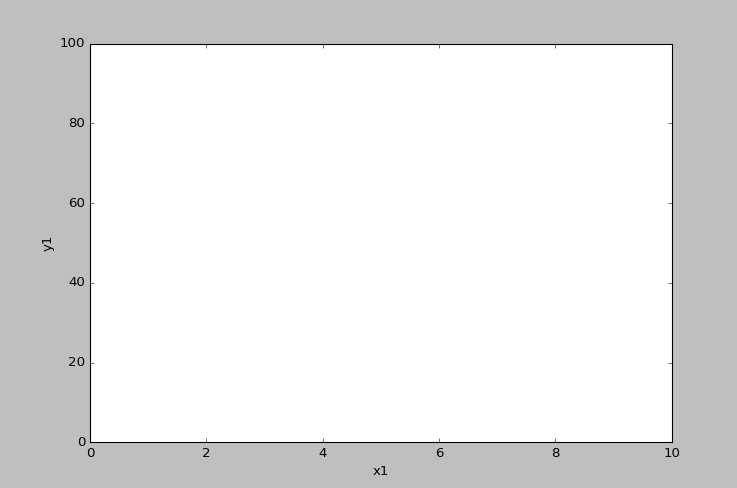到这一步还没有出现什么问题,但是当我将坐标系的x轴命名为"x轴"时,问题来了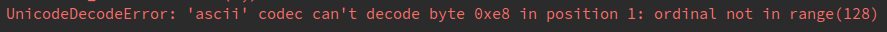这是一个常见的文件读取错误了,因为python读取文件时使用的编码默认是ascii而不是utf8,由此可以猜测matplotlib显示图表的流程可能是先临时保存为一个文件,再将这个文件读取出来,修改也很简单,将读取文件编码改为utf8即可
import sys
sys.setdefaultencoding('utf-8')
到这一步我以为问题可以解决了,但是事实证明我想的太简单了,显示出来是这样的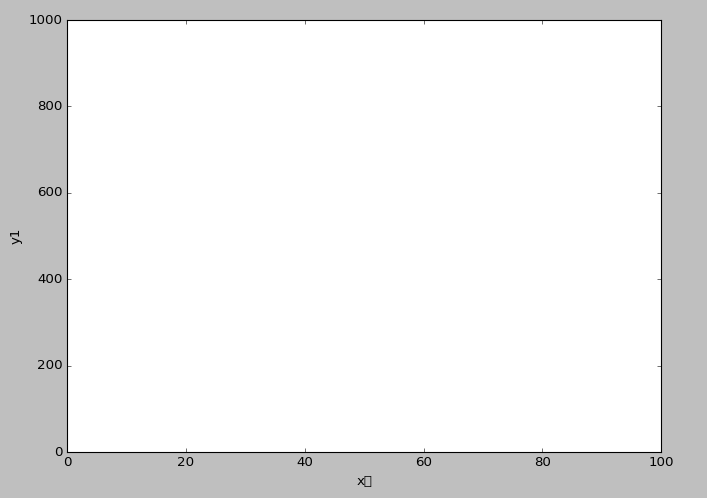(内心OS ： ？？？我的"轴"怎么变成方块了,几个意思？？？)
查阅了相关资料发现,原来matplotlib的图表显示并不支持中文,要显示中文可以使用plot中的rcParams函数,方法有很多种
from matplotlib import rcParams
from matplotlib.font_manager import FontProperties
myfont =  FontProperties(fname='/.../simhei.ttf',size=20) #读取本机上已有的中文字体文件并设置大小,此处设为"微软雅黑"
rcParams['axes.unicode_minus']=False #解决负号'-'显示为方块的问题

我这里采用局部显示,有输出中文的字段后对应要添加一串说明
plt.xlabel('x轴',fontproperties=myfont) #将坐标系x轴命名为x轴
带有中文字段的图表就构建成功了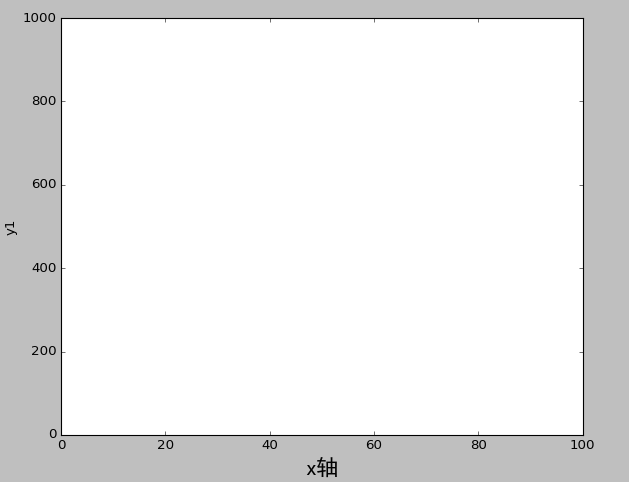到这一步,基本的框架已经没有说明显示的问题了,接下来我尝试输入数据,这一步我用到的主要为plot函数
x = np.linspace(1,100,20) #规定x区间范围为1,100,同时划分20份(5,10,15,20,25,....,100)
plt.plot(x,x*5,'or-')
#plt.plot(x,y,'format_string','**kwargs')
#plot单位为一组(x,y,format_string)
#format_string: 由颜色字符、风格字符和标记字符组成,顺序可随机
# 颜色字符：例 'b'蓝色      '#008000'RGB某颜色   '0.8'灰度值字符串
# 风格字符：例 '-'实线      '--'破折线           '-.'点划线；       '：'虚线
# 标记字符：例 '.'点标记    'o'实心圈             'v'倒三角         '^'上三角
#**kwargs: 更多组（x, y, format_string）

plt.show() #显示你的图表
输入数据后图表运行如图所示图表已经基本可以构建出来了,但是我想对这个图表加多一点信息,这里罗列一下pyplot中常用的几个文本显示函数
#文本显示函数
plt.xlabel('x轴',fontproperties=myfont,color='green') #将坐标系x轴命名为x1
plt.ylabel('y轴',fontproperties=myfont,color='blue') #将坐标系y轴命名为y1
plt.title('正相关函数 y=x*5',fontproperties=myfont,) #对整个图形进行命名
plt.text(40, 200, '节点1',fontproperties=myfont) #选择某个特殊节点进行说明
显示如下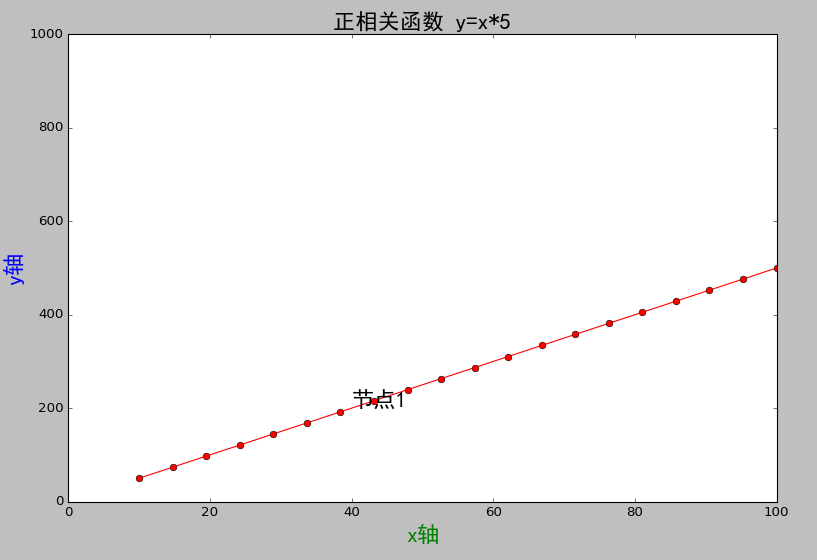matplotlib同时提供将多个图表显示在一个界面的功能,这里主要用到的函数为subplot2grid()
#划分区域绘图
plt.subplot2grid((2,2),(1,0),colspan=2,rowspan=1)
plt.subplot2grid((2,2),(0,0),colspan=1,rowspan=1)
plt.subplot2grid((2,2),(0,1),colspan=1,rowspan=1)
#plt.subplot2grid(GridSpec, CurSpec, colspan=1, rowspan=) 其中
#GridSpec为划分区域的数量
#CurSpec为选定的区域
#colspan为列数要延伸的区域
#rowspan为行数要延伸的区域
显示如下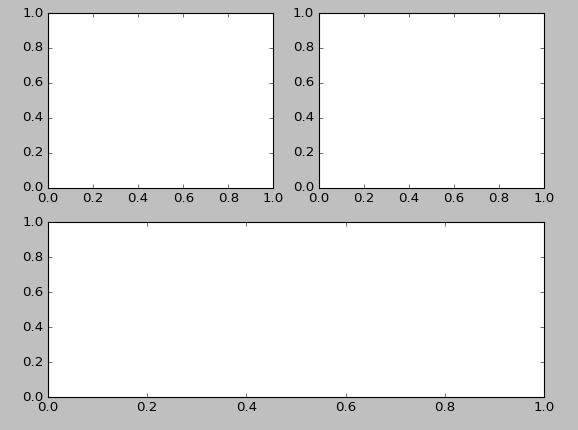以上的图表均为坐标图,但是实际生活中可能还要用到多种其他图形,比如饼图,散点图等等,以下为matplotlib的其他图表函数
#其他类型的图表
plt.plot(x,y , fmt) #坐标图
plt.bar(left, height, width, bottom) #条形图
plt.pie(data, explode) #饼图
plt.scatter(x, y) #散点图
plt.hist(x, bings, normed) #直方图
plt.boxplot(data, notch, position) #箱形图
plt.barh(width, bottom, left, height) #横向条形图
plt.polar(theta, r) #极坐标图
实操：用饼图,条形图,散点图和坐标图分别在同一界面绘制四张不同的表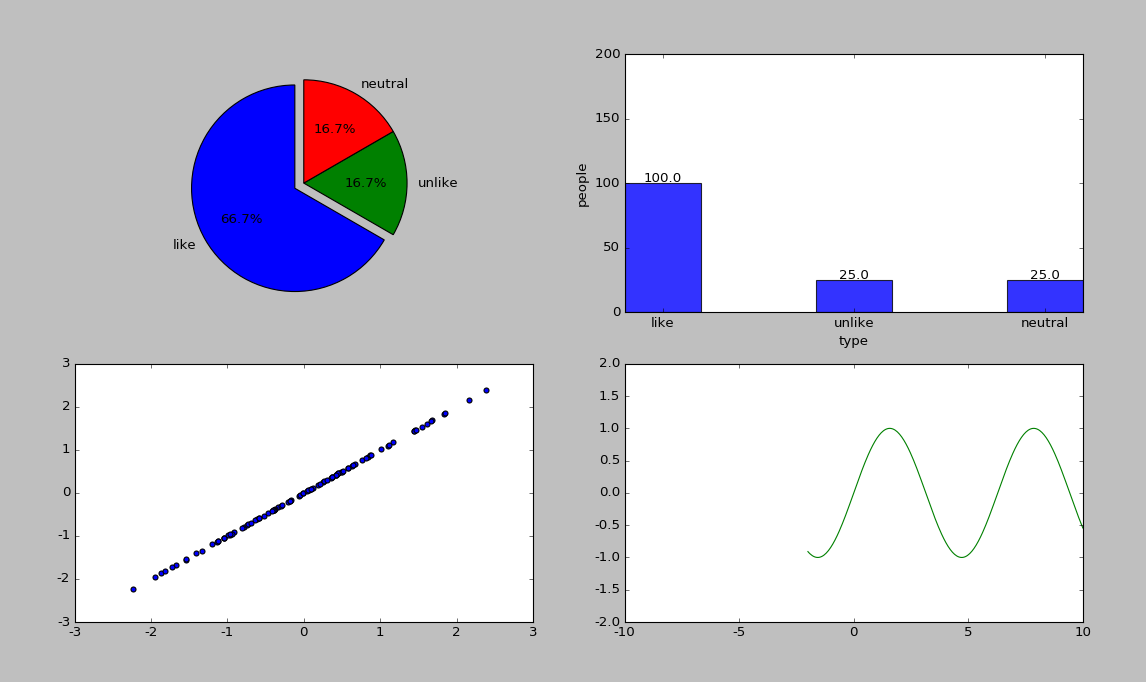#绘制饼图
#假设100个人里面有100个喜欢这款产品,25个不喜欢,25个中立
plt.subplot2grid((2,2),(0,0),rowspan=1,colspan=1)
lables = 'like','unlike','neutral' #设立三个区域标签
sizes = [100,25,25] #三个区域各自占比
explode = (0.1,0,0) #区域突出程度
plt.axis('equal') #转化为圆形图

#绘制条形图
#假设100个人里面有100个喜欢这款产品,25个不喜欢,25个中立
plt.subplot2grid((2,2),(0,1),rowspan=1,colspan=1)
labels = 'like','unlike','neutral' #设立横坐标三个标签
labels_num = [100,25,25]
x = range(len(labels_num))
plt.ylim(0,200) #纵坐标范围
plt.ylabel('people')
plt.xticks([index + 0.2 for index in x], labels)
plt.xlabel('type')
rects = plt.bar(left=x, height=labels_num, width=0.4, alpha=0.8, color='blue')
for rect in rects: #为每一条条形添加数字
height = rect.get_height() #依次读取每一条条形图的高度,与labels_num中的值相同
plt.text(rect.get_x() + rect.get_width() / 2, height+1, str(height), ha="center")

#绘制散点图
#100个数字中随机抽取100个绘制散点图
plt.subplot2grid((2,2),(1,0),rowspan=1,colspan=1)
random_num = np.random.randn(100)
plt.scatter(random_num,random_num)

#绘制坐标图
#绘制正弦函数
plt.subplot2grid((2,2),(1,1),rowspan=1,colspan=1)
plt.axis([-10,10,-2,2])
x=np.linspace(-2,10,256,endpoint=True)  #endpoint为刻度
plt.plot(x,np.sin(x),'g-')

plt.show()
以上就是我的matplotlib学习历程
展开全文python ubuntu
• https://matplotlib.org/stable/index.html ...matplotlib.pyplot是一个有命令风格的函数集合，它看起来和MATLAB很相似。每一个pyplot函数都使一副图像做出些许改变，例如创建一幅图，在图中创建一个绘图区域，在
编程字典Pandas教程 http://codingdict.com/article/8270 清华计算机博士带你学-Python金融量化分析 https://www.bilibili.com/video/BV1i741147LS?t https://matplotlib.org/stable/index.html
1. 介绍
matplotlib.pyplot是一个有命令风格的函数集合，它看起来和MATLAB很相似。每一个pyplot函数都使一副图像做出些许改变，例如创建一幅图，在图中创建一个绘图区域，在绘图区域中添加一条线等等。在matplotlib.pyplot中，各种状态通过函数调用保存起来，以便于可以随时跟踪像当前图像和绘图区域这样的东西。绘图函数是直接作用于当前axes（matplotlib中的专有名词，图形中组成部分，不是数学中的坐标系。）
2. plot函数
plot函数：绘制折线图
折线属性
线性linestyle（-，-.，–,…）点型marker（v，^，s，*，H，+，x，D，o，…）颜色color（b，g，r，y，k，w，…）
例子：
plt.plot([1,2,3,4,5],[4,5,6,1,3])
plt.show()

这表示的是(x,y)对，(1,4)(2,5)(3,6)(4,1)(5,3)。这里有第三个可选参数，它是字符串格式的，表示颜色和线的类型。该字符串格式中的字母和符号来自于MATLAB，它是颜色字符串和线的类型字符串的组合。默认情况下，该字符串参数是’b-‘，表示蓝色的实线。 举一个使用红色实心圆点画线绘制上述点集的例子：
plt.plot([1,2,3,4,5],[4,5,6,1,3],'-.ro')
plt.show()

多条曲线
与MATLAB一样可以使用figure命名一张图，并且作为分隔。
plt.figure('one')
a = np.arange(0,10,0.25)
plt.plot(a,a ** 2)
plt.figure('two')
plt.plot(a,a ** 2)
plt.plot(a,a ** 3)
plt.show()

工作在多图形(figures)和多坐标系(axes) MATLAB和pyplot都有当前图形(figure)和当前坐标系(axes)的概念。所有的绘图命令都是应用于当前坐标系的。gca()和gcf()(get current axes/figures)分别获取当前axes和figures的对象。通常，你不用担心这些，因为他们都在幕后被保存了，下面是一个例子，创建了两个子绘图区域(subplot)：
def f(t):
return np.exp(-t) * np.cos(2*np.pi*t)

t1 = np.arange(0.0, 5.0, 0.1)
t2 = np.arange(0.0, 5.0, 0.02)

plt.figure("2subplot")
plt.subplot(211)
plt.plot(t1, f(t1), 'bo', t2, f(t2), 'k')

plt.subplot(212)
plt.plot(t2, np.cos(2*np.pi*t2), 'r--')
plt.show()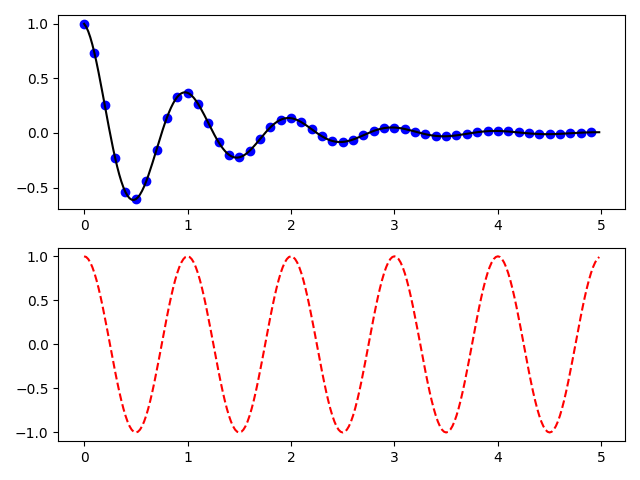figure()命令在这儿可以不写，因为figure(1)将会被默认执行，同样，subplot(111)也是默认被执行的。
subplot（）函数：subplot（numbRow ， numbCol ，plotNum ） or subplot(numbRow numbCol plotNum)，对。看清楚，可以不用逗号分开直接写在一起也是对的。
numbRow是plot图的行数；numbCol是plot图的列数；plotNum是指第几行第几列的第几幅图 ；
文字
text()命令可以被用来在任何位置添加文字，xlabel()、ylabel()、title()被用来在指定位置添加文字。
中文支持问题
python中使用matplotlib显示中文时,会遇到一些问题, 解决方案如下: 加入下面两行
plt.rcParams['font.sans-serif'] = ['SimHei']  # 用来正常显示中文标签
plt.rcParams['axes.unicode_minus'] = False  # 用来正常显示负号

常用字体：
黑体 SimHei 微软雅黑 Microsoft YaHei
微软正黑体 Microsoft JhengHei 新宋体 NSimSun
新细明体 PMingLiU 细明体 MingLiU
标楷体 DFKai-SB 仿宋 FangSong
楷体 KaiTi 仿宋_GB2312 FangSong_GB2312
楷体_GB2312 KaiTi_GB2312
图像标注
方法说明plt.title()设置图像标题plt.xlabel()设置x轴名称plt.ylabel()设置y轴名称plt.xlim()设置x轴范围plt.ylim()设置y轴范围plt.xticks()设置x轴刻度plt.yticks()设置y轴刻度plt.legend()设置曲线图例
例子：
import matplotlib.pyplot as plt
import numpy as np

plt.rcParams['font.sans-serif'] = ['SimHei']  # 用来正常显示中文标签
plt.rcParams['axes.unicode_minus'] = False  # 用来正常显示负号

# 设置数据
a = np.arange(0,5,0.1)

#设置输出的图片大小
figsize = 6.5,4.5
figure, ax = plt.subplots(figsize=figsize)

# 在同一幅图片上画两条折线
A,=plt.plot(a,a,'.g',label='y=x',linewidth=3.0)
B,=plt.plot(a,a ** 2,'-r',label='y=x^2',linewidth=3.0)
C,=plt.plot(a,a ** 3,'b-.',label='y=x^3',linewidth=3.0)
# B,=plt.plot(a,(3 * a ** 3 + 5*a**2+2*a+1),'b-.',label='B',linewidth=3.0)

# 设置图例并且设置图例的字体及大小
font1 = {'family': 'Times New Roman',
'weight': 'normal',
'size': 12,
}
legend = plt.legend(handles=[A, B, C], prop=font1)

# 设置坐标刻度值的大小以及刻度值的字体
plt.tick_params(labelsize=12)
labels = ax.get_xticklabels() + ax.get_yticklabels()
[label.set_fontname('Times New Roman') for label in labels]

# 设置横纵坐标的名称以及对应字体格式
font2 = {'family': 'FangSong',  # 仿宋
'weight': 'normal',
'size': 14,
}

plt.xlabel('横坐标',font2)
plt.ylabel('纵坐标',font2)
plt.title('Matplotlib实例——绘制数学函数图像',font2)

# 将文件保存至文件中并且画出图
plt.savefig('figure.eps')
plt.show()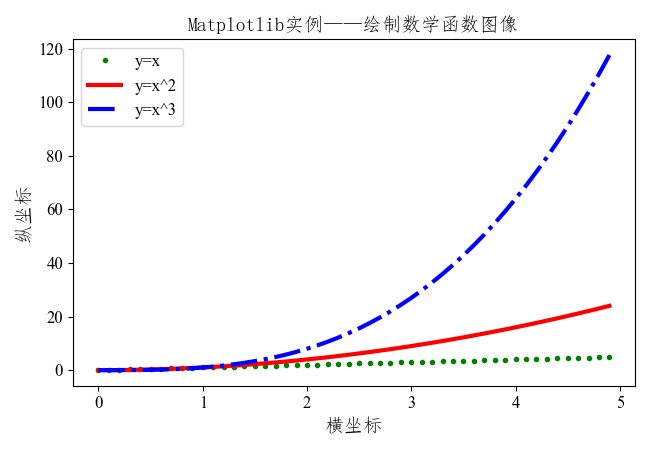展开全文python
• import matplotlib.pyplot as plt plt.rcParams['font.sans-serif'] = ['SimHei'] # 用来正常显示中文标签 plt.rcParams['axes.unicode_minus'] = False # 用来正常显示负号 p = data.plot(kind='kde', ...
• Plot函数：plot()的参数：plot(*args,**kwargs)，其中args为x，y以及绘图线的颜色和类型。matplotlib.lines.Line2D ¶class matplotlib.lines.Line2D（xdata，ydata，linewidth = None，linestyle = None，color =...
• ## python中matplotlib.pyplot使用简介

万次阅读 多人点赞 2018-02-27 20:13:11
pyplot介绍matplotlib.pyplot是一个有命令风格的函数集合，它看起来和MATLAB很相似。每一个pyplot函数都使一副图像做出些许改变，例如创建一幅图，在图中创建一个绘图区域，在绘图区域中添加一条线等等。在...
• 文章目录Matplotlib配置图例与颜色条配置图例默认情况下的图例图例外观设定指定loc参数设定图例位置指定frameon参数来设定边框指定ncol参数来设定标签列数指定圆角边框,增加边框阴影与改变边框透明度选择图例现实的...python 数据分析
• python -matplotlib figure操作 对于matplotlib当我们画图时，首先一步是对figure的定义，下面先给大家看一段代码 import matplotlib.pyplot as plt import numpy as np import os x=np.linspace(-3,3,50) y1=x*2+1...python
• import matplotlib.pyplot as plt import numpy as np x=np.linspace(0,1,50) y1=2*x+1 y2=x**2 plt.figure(num=1) #获取图片并命名 plt.plot(x,y1,color='blue',linewidth=1.0,linestyle='--',label='blue')
• 需要注意的是，多组数据的操作实际上都是通过Y来标记X的列，所以命名数据标示时，名字应该就是X矩阵中的每列的名称 plt . legend ( [ 'Column1' , 'Column2' . . . ] , loc = 6 , fontsize = 12 ) # 其中loc...
• 'c','d']) df.plot.hist(stacked=True, bins=20, colormap='Greens_r', alpha=0.5, grid=True) # 使用DataFrame.plot.hist()和Series.plot.hist()方法绘制 # stacked：是否堆叠 df.hist(bins=50) # 生成多个直方图python
• 本文实例讲述了python matplotlib模块基本图形绘制方法。分享给大家供大家参考，具体如下： matplotlib模块是python中一个强大的绘图模块 安装 pip install matplotlib 首先我们来画一个简单的图来感受它的神奇 ...
• Matplotlib文档链接：https://matplotlib.org/api/pyplot_summary.html Matplotlib中文文档：https://www.matplotlib.org.cn/ 实例参考：...
• 在Python学堂1-8中，我们通过一个实例（温湿度变化曲线对比分析图）对Matplotlib模块中的常用折线图进行了初步的认知学习。本章及后续几章内容是对前面内容的总结以及细化。系统性的带领大家共同学习Matplotlib中的...
• Python之Matplotlib库常用函数大全（含注释） 最近开始整理python的资料，博主建立了一个qq群，希望给大家提供一个交流的同平台78486745（点击进群）。 一般函数 plt.savefig(‘test’, dpi = 600) ：将绘制的图画...Python学习 Python函数 Python入门
• import matplotlib.pyplot as plt if __name__ == '__main__': X = np.linspace(-10, 10, 100) y_sin = np.sin(X) y_cos = np.cos(X) # 设置图像标题 plt.title('Comparison of sin(x) and cos(x)') # 设置...可视化 python 数据可视化
• plt.text(3.22,y1(3.2),'f(x)',fontsize=13)#文本 完整代码： import numpy as np import matplotlib.pyplot as plt #构造函数 def y1(x): return np.exp(-x)*np.cos(5*np.pi*x) plt.figure(figsize=(9,6)，)#图形...
• import matplotlib.pyplot as plt 画图命令 plt.plot() 括号内写参数，如plt.plot(x_list,y_list)，x_list,y_list是两个长度相同的list，分别代表plot图中各个点的横纵坐标，连起来就是plot图。 另外这里的plt...
• 许多场合下，我们需要将两条甚至多条曲线放置在一张图中进行对比，matplotlib可以轻松实现该要求。 完整代码如下： import matplotlib.pyplot as plt import numpy as np data = np.arange(0,1,0.01) #伪造从0到1以...python 可视化
• import matplotlib.pyplot as plt from matplotlib.font_manager import FontProperties font = FontProperties(fname=r"c:\windows\fonts\SimSun.ttc", size=14) plt.title(u"各等级的乘客年龄分布", ...python
• matplotlib库：是一个数据可视化的第三方库 使用pip命令安装pip install matplotlib 下载的速度堪忧 或者在这个官网上面下载：https://pypi.org/project/matplotlib/#files 选择好版本，下图为Python3.7 window64 ...python3.7
• 自动保存图表：pyplot.savefig('D:\... 1 # 使用matplotlib.pyplot.scatter绘制散点 2 import matplotlib.pyplot as plt 3 from pylab import mpl 4 5 # 设置默认字体，解决中文显示乱码问题 6 mpl.rcPar...
• 因此使用matplotlib里的patches模块，就可以去掉这些担忧。 在学习这个新模块时，先来一个简单的例子，比如画一个圆如下： 画这个圆时，调用了函数mpatches.Circle（），它是来自模块matplotlib.patches，通过...python
• ## Python基础教程，Python入门教程

万次阅读 多人点赞 2019-07-15 10:23:21
Python Matplotlib plot 3. Python Matplotlib legend 4. Python Matplotlib time、xlabel、ylabel、xticks、yticks 5. Python Matplotlib subplot 6. Python Matplotlib pie 7. Python Matplotlib...python
• 在实践中，经常需要在一张画布上绘制多个图形，subplot 函数便是 matplotlib 的 pyplot 模块中用于绘制多图的函数。其调用格式如下： subplot(nrows, ncols, index, **kwargs) subplot(pos, **kwargs) subplot(ax) ...python 数据可视化 subplot
• A picture says a thousand words, and with Python’s matplotlib library, it fortunately takes far less than a thousand words of code to create a production-quality graphic. 一幅图片说出一千个单词，而...可视化 python java 大数据 人工智能
• Matplotlib 教程 本文为译文，原文载于此，译文原载于此。本文欢迎转载，但请保留本段文字，尊重作者和译者的权益。谢谢。: ) 介绍 Matplotlib 可能是 Python 2D-绘图领域使用最广泛的套件。它能让使用者很...
• Matplotlib：可视化颜色命名分类和映射颜色分类 python+matplotlib绘图线条类型、颜色、散点类型 python的matplotlib画图库参考自matlab，但是功能更加强大，色标更加丰富，颜色更加自然，线条更加流畅。 matlab与...python matlab...